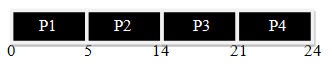• CPU scheduling deals with the problem of deciding which of each process in the ready queue is to be allocated to the CPU.
• There are many algorithms available for scheduling purpose each with its own advantages and disadvantages as well. CPU scheduling algorithms are important to make the effective use of CPU time.
• Some most common CPU scheduling algorithms are as follow.
1. First Come First Serve (FCFS)
2. Shortest Job First (SJF)
3. Priority Scheduling
4. Round – Robin Scheduling
• In this article, we are going to discuss First Come First Serve CPU scheduling algorithm in detail.

## First Come First Serve (FCFS)

• It is the simplest CPU scheduling algorithm. According to this scheme, a first process that request to CPU first is allocated the CPU first based on the rule “first come first serve”. The implementation of FCFS policy is easily managed by FIFO queue. When a process enters into the ready queue, its PCB is linked at the end of the queue.
• When the CPU is free, it is allocated to the process at the head of queue.
• Let us see first come first serve algorithm at work by the following example:

Consider the following processes and their CPU burst time and find out average waiting time and average turn around time using FCFS CPU scheduling algorithm. Note that processes are given in their arrival order.

Process Burst Time (Mils)
P1 5
P2 9
P3 7
P4 3
• According to First Come First Serve policy the process that comes first will be allocated the CPU first.
• In our example, the first process is the process P1 with the burst time of 5 millisecond so process P1 will get the CPU first. Since P1 is the first process, waiting time for P1 will be zero.
• Process P2 will get the CPU next but it has to wait till the process P1 gets completed i.e. five milliseconds. Process P1 will execute for 5 milliseconds so waiting time for process P2 will be five milliseconds, after that P3 wiil get the CPU and so on.
• So first of all we need to prepare gent chart to represent each process as follow:• Waiting Time of the Processes will be as follow:
``P1: -> 0, P2 -> 5, P3 -> 14 (5+9), P4 -> 21 (5+9+7)``

Average Waiting Time = Total Time / No.of Process

``````
= 0 + 5 + 14 + 21

= 40 / 4

= 10 Mills.``````

Average Turnaround Time = Total Turnaround Time / No.of Process

``````P1 -> 5 , P2 -> 14, P3 -> 21, P4 -> 24

= 5 + 14 + 21 + 24

= 64 / 4

= 16 Mills.``````
• A CPU burst time varies, and average waiting time may also vary. It is non- preemptive scheduling scheme.
• Once the CPU has been allocated to the memory, the process keeps the CPU until it releases the CPU, either by terminating or by requesting input/output.